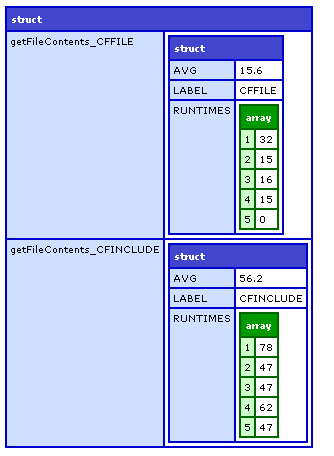Which is Faster?

In programming, discussions often come up about which approach to solving a problem is faster. Although I think these discussions often miss the point (as other decision factors often trump execution speed), they are still often informative.

I have had a few occassions recently where I wanted to answer these questions myself. It seemed tedious to continue to write code to test that out, so I decided to write a generic "Code Timer" to run code and test for execution times.

Here is CodeTimer.cfc:

<cfcomponent>

<cffunction name="init" access="public" returntype="any" output="false" hint="I initialize CodeTimer and set any arguments given to me as variables in the component.">

<cfset StructAppend(variables,arguments)>

<cfreturn This>
</cffunction>

<cffunction name="runCodeTest" access="public" returntype="any" output="false" hint="I run any functions given to me.">
<cfargument name="sets" type="numeric" default="100" hint="The number of sets of code tests to run. Each set will be timed.">
<cfargument name="reps" type="numeric" default="10" hint="The number of repetitions to run each test per set. The time to execute the whole set, not an individual repetition will be timed.">

<cfset var ii = 0>
<cfset var jj = 0>
<cfset var sResult = StructNew()>
<cfset var sMethod = 0>

<cfset var method = 0>
<cfset var start = 0>
<cfset var end = 0>

<cfloop collection="#arguments#" item="arg">
<!--- Only test out functions --->
<cfif IsCustomFunction(arguments[arg])>
<!--- Have to copy method to interrim variable to use it --->
<cfset method = arguments[arg]>
<cfset sMethod = getMetadata(method)>
<!--- Only test out functions with no arguments --->
<cfif NOT ArrayLen(sMethod.Parameters)>
<cfset sResult[sMethod.name] = StructNew()>
<!--- Make a label for the results --->
<cfif StructKeyExists(sMethod,"DisplayName")>
<cfset sResult[sMethod.name].Label = sMethod.DisplayName>
<cfelse>
<cfset sResult[sMethod.name].Label = sMethod.Name>
</cfif>
<!--- Run that code! --->
<cfset sResult[sMethod.name].RunTimes = ArrayNew(1)>
<cfloop index="ii" from="1" to="#arguments.sets#" step="1">
<cfset start = getTickCount()>
<cfloop index="jj" from="1" to="#arguments.reps#" step="1">
<cfset method()>
</cfloop>
<cfset end = getTickCount()>
<cfset ArrayAppend(sResult[sMethod.name].RunTimes,(end-start))>
</cfloop>
<cfset sResult[sMethod.name].avg = ArrayAvg(sResult[sMethod.name].RunTimes)>
</cfif>
</cfif>
</cfloop>

<cfreturn sResult>
</cffunction>

</cfcomponent>

My most recent curiosity was about how to load the contents of a file as a variable. I could use CFFILE or use CFSAVECONTENT and CFINCLUDE.

Here are my test functions:

<cffunction name="getFileContents_CFFILE" displayname="CFFILE">
<cfset var result = "">

<cffile action="read" file="#filepath#" variable="result">

<cfreturn result>
</cffunction>
<cffunction name="getFileContents_CFINCLUDE" displayname="CFINCLUDE">
<cfset var result = "">

<cfsavecontent variable="result"><cfinclude template="#filename#"></cfsavecontent>

<cfreturn result>
</cffunction>

Note that these functions are not well encapsulated. They each require an outside variable. So, I need to make sure those variables exist in CodeTimer. Here is how I initialize CodeTimer:

<cfset filename = "tests/short.txt">
<cfset oRunner = CreateObject("component","CodeTimer").init(filename=filename,filepath=ExpandPath(filename))>

Now the "filename" and "filepath" variables exist in CodeTimer.

The only thing left to do is run the code and get the results:

<cfset result = oRunner.runCodeTest(100,5,getFileContents_CFFILE,getFileContents_CFINCLUDE)>
<cfdump var="#result#">

The first argument is the number of sets of repetitions. The second argument is the number of repetitions per set. Each set is time, but each rep is not. The sets/reps paradigm was inspired by Ben Nadel (the master of scientific testing in ColdFusion) and his interest in exercise and I think it works pretty well.

If you are interested, CFFILE consistently outperforms CFSAVECONTENT and CFINCLUDE for both short (5 lines) and long (500 lines) files. I actually expected CFSAVECONTENT and CFINCLUDE to slightly outperform CFFILE so this was definitely a useful find for me.

Here is the final test:

<cffunction name="getFileContents_CFFILE" displayname="CFFILE">
<cfset var result = "">

<cffile action="read" file="#filepath#" variable="result">

<cfreturn result>
</cffunction>
<cffunction name="getFileContents_CFINCLUDE" displayname="CFINCLUDE">
<cfset var result = "">

<cfsavecontent variable="result"><cfinclude template="#filename#"></cfsavecontent>

<cfreturn result>
</cffunction>

<cfset filename = "tests/long.txt">
<cfset oTimer = CreateObject("component","CodeTimer").init(filename=filename,filepath=ExpandPath(filename))>

<cfset result = oTimer.runCodeTest(5,100,getFileContents_CFFILE,getFileContents_CFINCLUDE)>
<cfdump var="#result#">Now any time I want to compare code execution time, I can just wrap up each option into a function and test it out.

Comments (Comment Moderation is enabled. Your comment will not appear until approved.)
Thanks for sharing this Steve. This will be a handy addition to my toolbox.
# Posted By Tony Garcia | 8/7/09 7:10 AM
BlogCFC was created by Raymond Camden. This blog is running version 5.8.001.